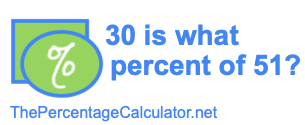30 is what percent of 51?When you ask "30 is what percent of 51?" you are asking what share per hundred (percent) 30 is of 51 (30 is what % of 51?). To show you how to calculate the answer we start by displaying the percent formula below:

 Whole × Percent 100
=  Part

In the problem "30 is what percent of 51?", 30 is the Part, 51 is the Whole, and Percent is what we are solving. When we enter the Whole and the Part in our formula, we get this:

 51 × Percent 100
=  30

To solve for Percent, we first multiply both sides by 100 to get rid of the denominator on the left side:

 51 × Percent × 100 100
=  30 × 100

 51 × Percent
=  3000

Next, we divide both sides by 51 to get Percent by itself on the left side:

 51 × Percent 51
=
 3000 51

Percent  =
 3000 51

That's it! Above is the fractional answer to "30 is what percent of 51?". To get the decimal answer, you simply divide the numerator by the denominator. Thus, here is the final answer to "30 is what percent of 51?" in decimal percent form:

3000/51
= 58.8235294117647
≈ 58.8235%

Is What Percent of Calculator
Here you can submit another problem for us to solve:

is what percent of

30 is what percent of 52?
Want to learn more? Here is the next problem on our list.